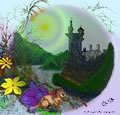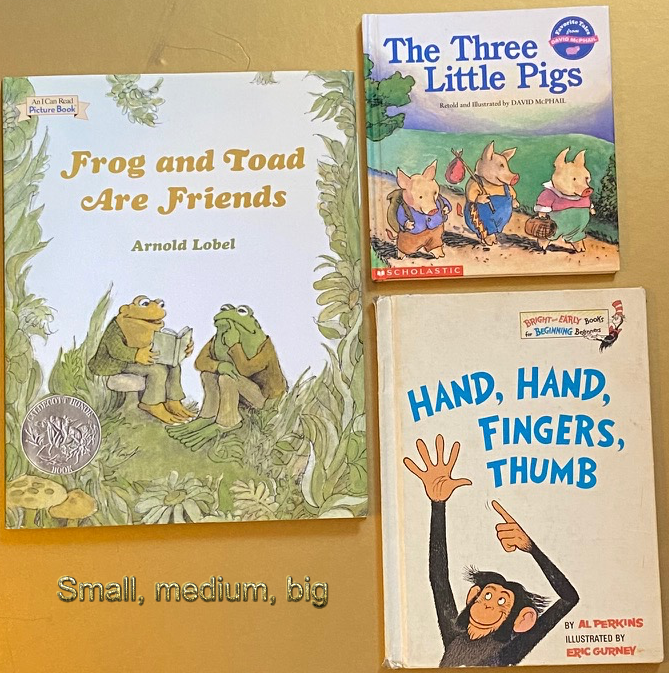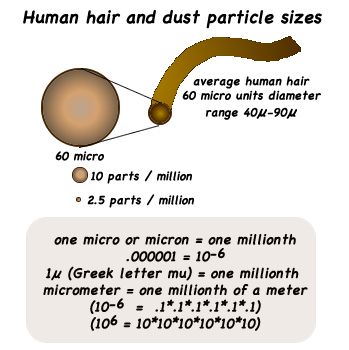# Measurement

## Knowledge base & tools to develop mathematical literacy

### Knowledge base

The mathematical knowledge base includes concepts necessary for learners to conceptualize to become mathemtically literate and misconceptions that must be over come. Particularly important for measurement include:

### Information to facilitate literacy in  measurement & tools to achieve it (pedagogy)

Historical weights found around the world suggest traders recognized the need for standard measurements.### Activities to facilitate measurement literacy

•Introductory activities. Have learners directly compare objects as big and little. Compare hands, clothes, bowls, eating utensils, toys, crayons, blocks, and more. Then have them directly compare and order three objects as big, medium, & small. Work towards sequencing larger sets of objects.
• Metric fact sheet - Fact sheet with metric units: meter, liter, gram, prefixes on a number line, diagram of dust particle & human hair, metric prefixes with symbols to + & - powers of thirty.
• Measurement - primary level introduction to measurement with first straw activity for to standard units of linear measurement, then similar hands on measurement activities for volume, mass, & temperature
• Measurement: middle level review of linear, mass, volume, measurement of matter, with an introduction to density with directed inquiry activities & blank student lab notes
• Observation & measurement - How do we observe? properties to observe, change, measurement, properties to measure, & measurement procedure work sheet
• Density - learning cycle inquiry activities for middle grade to apply measurement: linear, mass, volume, of matter to calculate density of solids, liquids, & gases. Includes 14 activities with lesson plans & lab sheets.
• Cartography map making, angle measurement, compass, clinometer, measure height, measure distance, locating objects with distance & angle combinations, contour maps,
• Measuring heights of trees & other objects - clinometer, shadows, scale (Biltmore) stick
• 21 Centimeter card game: make a deck with 45 cards. Draw a slanted straight line, the following lengths, on each card. Slant the lines on each card at different degrees. Make:
• 4 cards each with a - 1 cm line,
• 4 cards each with a - 2 cm line,
• 4 cards each with a - 3 cm line,
• 4 cards each with a - 4 cm line,
• 4 cards each with a - 5 cm line,
• 5 cards each with a - 6 cm line,
• 5 cards each with a - 7cm line,
• 5 cards each with a - 8 cm line,
• 5 cards each with a - 9 cm line, &
• 5 cards each with a - 10cm line.
Game play: Deal three cards to each player and play like 21. Player that line lengths add closest to 21 cm, without going over is the winner. After the deal each player can choose to be dealt another card or hold. Player closest to 21 cm, with out going over wins. Winner measures each card with a cm ruler to verify their score.
Alternative version: Play as above and record the lengths of their cards, if they don't go over 21. First player to 121 wins.
• Linear & volume -
• Temperature How does temperature in centigrade Celsius compare to temperature in Fahrenheit? Facts: Water freezes at 32 degrees Fahrenheit and 0 degrees Celsius. Water boils at 212 degrees Fahrenheit and 100 degrees Celsius. Find a thermometer that has both scales and read from one scale to the other. C = (F - 32) * 5/9; C = (F - 32) / 9/5; F = (C * 9/5) + 32 Verify or prove the formulas.
• Money - Sequence of outcomes to develop with activities to learn to count change:
• Know people use money to buy goods and services.
• Sort money by its appearance.
• Draw pictures of coins and paper money.
• Select coins on their desk (penny, quarters, dimes and nickel) as called.
• Identify and state the values of money (penny = 1 cent , quarter = 25 cents, dime = 10 cents, nickel = 5 cents, ... ).
• Sort money by its value.
• Order money by its value.
• Recognize different coins can have similar values.
• Represent coins and their values: draw a circle for each coin (penny, quarter, dime, nickel) with the value inside: (25, 25, 10, 10, 5).
• Identify a coin, its value, and point to its value represented on a number dot sequence, trail, or line. Select a second coin. Identify the coin, its value, and show how to add and represent its value and sum of both.
• Identify a coin, its value, and point to its value represented on a labeled hundreds chart. Select a second coin. Identify the coin, its value, and show how to add and represent its value and sum of both. Draw an arrow from 1 to the value of the first coin. Circle the value and draw an arrow for the value of the next coin and circle the sum. (arrow math)
• Use arrow math and a labeled hundreds chart chart to solve coin problems with two addends.
• Use arrow math and a labeled hundreds chart chart to solve coin problems with more than two addends.
• Given an assorted collection of coins (that add to less than 100 cents), Use arrow math and a labeled hundreds chart chart to solve coin problems with more than two addends.
• Identify different orders to count change more efficiently. (Start with the largest coins, group similar coins, group by easy numbers (10, 50, 100, & 25).
• Repeat the above procedures on a blank hundreds chart.
• Repeat the above procedures with paper money.

Can use the counting money strategy for counting decimal numbers. Write the starting value (1.89), select a count (.01), write it, write the sum (1.90), write the next coin (.10), then the sum (2.00), and continue as before.

Other development & activities pages:

Process dimensions:

Content dimensions:Measurement is never exact.

It is only as accurate as who and with what is doing the measurement.